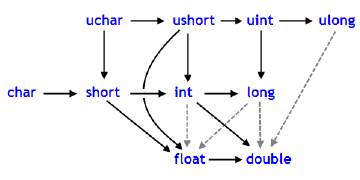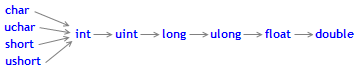﻿ 类型转换 - 数据类型 - 语言基础 - MQL4参考

MQL4参考语言基础数据类型类型转换

# Typecasting

### Casting Numeric Types

Often a necessity occurs to convert one numeric type into another. Not all numeric types can be converted into another. Here is the scheme of allowed casting:Solid lines with arrows indicate changes that are performed almost without any loss of information. Instead of the char type, the bool type can be used (both take 1 byte of memory), instead of type int, the color type can be used (4 bytes), instead of the long type, datetime can be used (take 8 bytes). The four dashed grey lines, also arrowed, denote conversions, when the loss of precision can occur. For example, the number of digits in an integer equal to 123456789 (int) is higher than the number of digits that can be represented by float.

 int n=123456789;    float f=n;    // the content of f is equal to 1.234567892E8    Print("n = ",n,"   f = ",f);    // result n= 123456789    f= 123456792.00000

A number converted into float has the same order, but is less accurate. Conversions, contrary to black arrows, can be performed with possible data loss. Conversions between char and uchar, short and ushort, int and uint, long and ulong (conversions to both sides), may lead to the loss of data.

As a result of converting floating point values to integer type, the fractional part is always deleted. If you want to round off a float to the nearest whole number (which in many cases is more useful), you should use MathRound().

Example:

 //--- Gravitational acceleration    double g=9.8;    double round_g=(int)g;    double math_round_g=MathRound(g);    Print("round_g = ",round_g);    Print("math_round_g = ",math_round_g); /*    Result:    round_g = 9    math_round_g = 10 */

If two values are combined by a binary operator, before the operation execution the operand of a lower type is converted to the higher type in accordance with the priority given in the below scheme:The data types char, uchar, short, and ushort unconditionally are converted to the int type.

Examples:

 char   c1=3; //--- First example    double d2=c1/2+0.3;    Print("c1/2 + 0.3 = ",d2); // Result:   c1/2+0.3 = 1.3   //--- Second example    d2=c1/2.0+0.3;    Print("c1/2.0 + 0.3 = ",d2); // Result:   c1/2.0+0.3 = 1.8

The calculated expression consists of two operations. In the first example, the variable c1 of the char type is converted to a temporary variable of the int type, because the second operand in the division operation, the constant 2, is of the higher type int. As a result of the integer division 3/2 we get the value 1, which is of the int type.

In the second operation of the first example, the second operand is the constant 0.3, which is of the double type, so the result of the first operation is converted into a temporary variable of the double type with a value of 1.0.

In the second example the variable of the char type c1 is converted to a temporary variable of the double type, because the second operand in the division operation, the constant 2.0, is of the double type; no further conversions are made.

### Typecasting of Numeric Types

In the expressions of the MQL4 language both explicit and implicit typecasting can be used. The explicit typecasting is written as follows:

 var_1 = (type)var_2;

An expression or function execution result can be used as the var_2 variable. The function style notation of the explicit typecasting is also possible:

 var_1 = type(var_2);

Let's consider an explicit typecasting on the basis of the first example.

 //--- Third example    double d2=(double)c1/2+0.3;    Print("(double)c1/2 + 0.3 = ",d2); // Result:   (double)c1/2+0.3 = 1.80000000

Before the division operation is performed, the c1 variable is explicitly cast to the double type. Now the integer constant 2 is cast to the value 2.0 of the double type, because as a result of converting the first operand has taken the double type. In fact, the explicit typecasting is a unary operation.

Besides, when trying to cast types, the result may go beyond the permissible range. In this case, the truncation occurs. For example:

 char c;    uchar u;    c=400;    u=400;    Print("c = ",c); // Result c=-112    Print("u = ",u); // Result u=144

Before operations (except for the assignment ones) are performed, the data are converted into the maximum priority type. Before assignment operations are performed, the data are cast into the target type.

Examples:

 int    i=1/2;        // no types casting, the result is 0    Print("i = 1/2  ",i);      int k=1/2.0;         // the expression is cast to the double type,    Print("k = 1/2  ",k);  // then is to the target type of int, the result is 0      double d=1.0/2.0;    // no types casting, the result is 0.5    Print("d = 1/2.0; ",d);      double e=1/2.0;      // the expression is cast to the double type,    Print("e = 1/2.0; ",e);// that is the same as the target type, the result is 0.5      double x=1/2;        // the expression of the int type is cast to the double target typr,    Print("x = 1/2; ",x);  // the result is 0.0

When converting  long/ulong type into double, precision may be lost in case the integer value is greater than 9223372036854774784 or less than -9223372036854774784.

 void OnStart()   {    long l_max=LONG_MAX;    long l_min=LONG_MIN+1; //--- define the highest integer value, which does not lose accuracy when being cast to double    while(l_max!=long((double)l_max))       l_max--; //--- define the lowest integer value, which does not lose accuracy when being cast to double    while(l_min!=long((double)l_min))       l_min++; //--- derive the found interval for integer values      PrintFormat("When casting an integer value to double, it must be "                "within [%I64d, %I64d] interval",l_min,l_max); //--- now, let's see what happens if the value falls out of this interval    PrintFormat("l_max+1=%I64d, double(l_max+1)=%.f, ulong(double(l_max+1))=%I64d",                l_max+1,double(l_max+1),long(double(l_max+1)));    PrintFormat("l_min-1=%I64d, double(l_min-1)=%.f, ulong(double(l_min-1))=%I64d",                l_min-1,double(l_min-1),long(double(l_min-1))); //--- receive the following result // When casting an integer value to double, it should be within [-9223372036854774784, 9223372036854774784] interval // l_max+1=9223372036854774785, double(l_max+1)=9223372036854774800, ulong(double(l_max+1))=9223372036854774784 // l_min-1=-9223372036854774785, double(l_min-1)=-9223372036854774800, ulong(double(l_min-1))=-9223372036854774784   }

### Typecasting for the String Type

The string type has the highest priority among simple types. Therefore, if one of operands of an operation is of the string type, the second operand will be cast to a string automatically. Note that for a string, a single dyadic two-place operation of addition is possible. The explicit casting of string to any numeric type is allowed.

Examples:

 string s1=1.0/8;            // the expression is cast to the double type,    Print("s1 = 1.0/8; ",s1);     //  then is to the target type of string, // result is "0.12500000" (a string containing 10 characters)      string s2=NULL;             // string deinitialization    Print("s2 = NULL; ",s2);      // the result is an empty string    string s3="Ticket N"+12345; // the expression is cast to the string type    Print("s3 = \"Ticket N\"+12345",s3);      string str1="true";    string str2="0,255,0";    string str3="2009.06.01";    string str4="1.2345e2";    Print(bool(str1));    Print(color(str2));    Print(datetime(str3));    Print(double(str4));

### Typecasting of Simple Structure Types

Data of the simple structures type can be assigned to each other only if all the members of both structures are of numeric types. In this case both operands of the assignment operation (left and right) must be of the structures type. The member-wise casting is not performed, a simple copying is done. If the structures are of different sizes, the number of bytes of the smaller size is copied. Thus the absence of union in MQL4 is compensated.

Examples:

 struct str1   {    double  d;   }; //--- struct str2   {    long    l;   }; //--- struct str3   {    int     low_part;    int     high_part;   }; //--- struct str4   {    string  s;   }; //+------------------------------------------------------------------+ void OnStart()   {    str1 s1;    str2 s2;    str3 s3;    str4 s4; //---    s1.d=MathArcsin(2.0);        // get the invalid number -1. # IND    s2=s1;    printf("1.  %f    %I64X",s1.d,s2.l); //---    s3=s2;    printf("2.  high part of long %.8X   low part of long %.8X",           s3.high_part,s3.low_part); //---    s4.s="some constant string";    s3=s4;    printf("3.  buffer len is %d   constant string address is 0x%.8X",           s3.low_part,s3.high_part);   }

Another example illustrates the method of organizing a custom function for receiving RGB (Red, Green, Blue) representation from the color type. Create two structures of the same size but with different contents. For convenience, let's add a function returning the RGB representation of a color as a string.

 #property script_show_inputs input color          testColor=clrBlue;// set color for testing //--- structure for representing color as RGB struct RGB   {    uchar             blue;          // blue component of color    uchar             green;         // green component of color    uchar             red;           // red component of color    uchar             empty;         // this byte is not used    string            toString();    // function for receiving a string   }; //--- function for showing color as a string string RGB::toString(void)   {    string out="("+(string)red+":"+(string)green+":"+(string)blue+")";    return out;   } //--- structure for storing of the built-in color type struct builtColor   {    color             c;   }; //+------------------------------------------------------------------+ //| Script program start function                                    | //+------------------------------------------------------------------+ void OnStart()   { //--- a variable for storing in RGB    RGB colorRGB; //--- variable for storing the color type    builtColor test;    test.c=testColor; //--- casting two structures by copying contents    colorRGB=test;    Print("color ",test.c,"=",colorRGB.toString()); //---   }

### Typecasting of Base Class Pointers to Pointers of Derivative Classes

Objects of the open generated class can also be viewed as objects of the corresponding base class. This leads to some interesting consequences. For example, despite the fact that objects of different classes, generated by a single base class, may differ significantly from each other, we can create a linked list (List) of them, as we view them as objects of the base type. But the converse is not true: the base class objects are not automatically objects of a derived class.

You can use the explicit casting to convert the base class pointers to the pointers of a derived class. But you must be fully confident in the admissibility of such a transformation, because otherwise a critical runtime error will occur and the mql4 program will be stopped.

### Dynamic typecasting using dynamic_cast operator

Dynamic typecasting is performed using dynamic_cast operator that can be applied only to pointers to classes. Type validation is performed at runtime. This means that the compiler does not check the data type applied for typecasting when dynamic_cast operator is used. If a pointer is converted to a data type which is not the actual type of an object, the result is NULL.

 dynamic_cast  ( expression )

The type-id parameter in angle brackets should point to a previously defined class type. Unlike C++, expression operand type can be of any value except for void.

Example:

 class CBar { }; class CFoo : public CBar { };   void OnStart()   {    CBar bar;     //--- dynamic casting of *bar pointer type to *foo pointer is allowed    CFoo *foo = dynamic_cast(&bar); // no critical error       Print(foo);                             // foo=NULL       //--- an attempt to explicitly cast a Bar type object reference to a Foo type object is forbidden    foo=(CFoo *)&bar;                       // critical runtime error    Print(foo);                             // this string is not executed   }动态数组目标空型和NULL常量